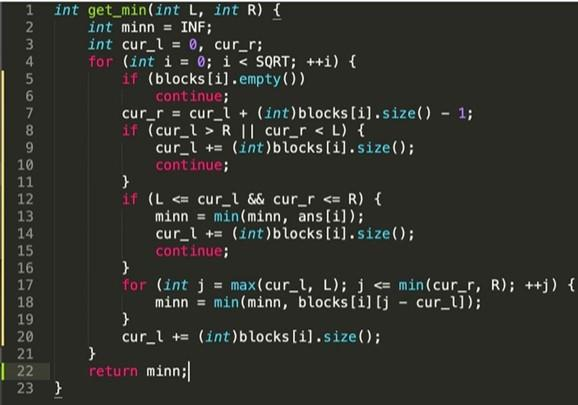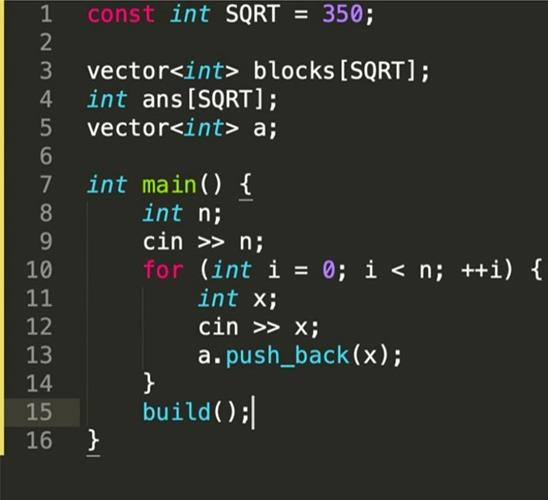Loading, please wait...# SQRT-decomposition in sort | data structure

May 05, 2020 data structure, Algorithms, DS, 4149 Views
It’s more, than programming.. it’s my fav data structure

It’s more than programming... it’s my fav data structure❤️

# SQRT-decomposition!

1. Helps you to insert, erase element with complexity O(sqrt(n))

2. Answer to different queries (min/max on the segment of array, sum, etc) with complexity O(sqrt(n)).

3. You can answer more complicated queries (for example, count of numbers, which are no greater thank on the segment) with O(sqrt(n)log(n)) complexity
How does it work?

## Let's See:

1. We divide array (vector<int> a) on blocks (vector<int> blocks[SQRT]) with length SQRT. For every block, we count answer (int ans[SQRT]). Swipe to see the initialization and build() function.2. If we need to insert (function add()) a number (int x) to position (int pos), we just save cur_l, cur_r - current indexes for block number i and check, if cur_l <= pos && pos <= cur_r. If it’s true, then we simply insert the element to the vector blocks[i] and recount the answer (ans[i])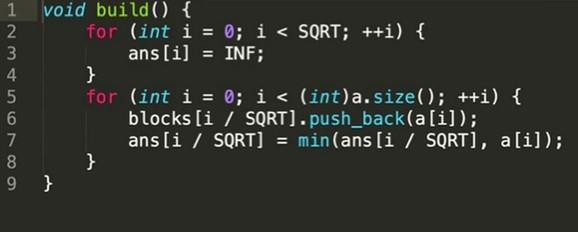3. Erase function del() works in the same way.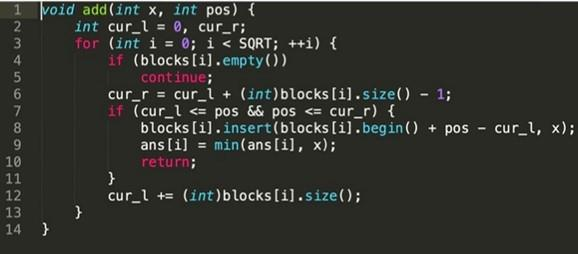4. If we need to answer the query (minimum on the segment L; R), we just check every block, if its indexes are between L and R, and then recount the answer Minn.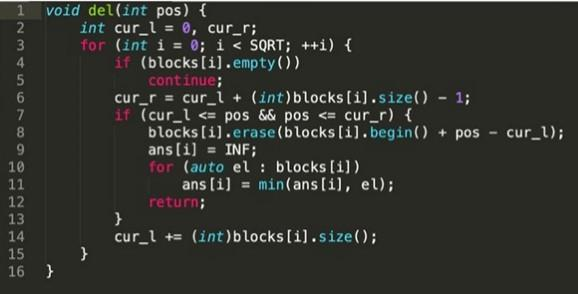5. We need to rebuild our structure every sqrt(Q) times (where Q is the number of queries) because the size of one block can become too big.
What is your favorite data structure❓